Linear Momentum and Collisions

# Linear Momentum and Force

OpenStaxCollege

### Learning Objectives

• Define linear momentum.
• Explain the relationship between momentum and force.
• State Newton’s second law of motion in terms of momentum.
• Calculate momentum given mass and velocity.

# Linear Momentum

The scientific definition of linear momentum is consistent with most people’s intuitive understanding of momentum: a large, fast-moving object has greater momentum than a smaller, slower object. Linear momentum is defined as the product of a system’s mass multiplied by its velocity. In symbols, linear momentum is expressed as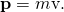Momentum is directly proportional to the object’s mass and also its velocity. Thus the greater an object’s mass or the greater its velocity, the greater its momentum. Momentum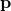is a vector having the same direction as the velocity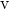. The SI unit for momentum is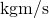.

Linear Momentum

Linear momentum is defined as the product of a system’s mass multiplied by its velocity:Calculating Momentum: A Football Player and a Football

(a) Calculate the momentum of a 110-kg football player running at 8.00 m/s. (b) Compare the player’s momentum with the momentum of a hard-thrown 0.410-kg football that has a speed of 25.0 m/s.

Strategy

No information is given regarding direction, and so we can calculate only the magnitude of the momentum,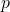. (As usual, a symbol that is in italics is a magnitude, whereas one that is italicized, boldfaced, and has an arrow is a vector.) In both parts of this example, the magnitude of momentum can be calculated directly from the definition of momentum given in the equation, which becomeswhen only magnitudes are considered.

Solution for (a)

To determine the momentum of the player, substitute the known values for the player’s mass and speed into the equation.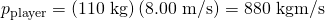Solution for (b)

To determine the momentum of the ball, substitute the known values for the ball’s mass and speed into the equation.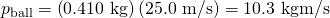The ratio of the player’s momentum to that of the ball is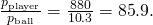Discussion

Although the ball has greater velocity, the player has a much greater mass. Thus the momentum of the player is much greater than the momentum of the football, as you might guess. As a result, the player’s motion is only slightly affected if he catches the ball. We shall quantify what happens in such collisions in terms of momentum in later sections.

# Momentum and Newton’s Second Law

The importance of momentum, unlike the importance of energy, was recognized early in the development of classical physics. Momentum was deemed so important that it was called the “quantity of motion.” Newton actually stated his second law of motion in terms of momentum: The net external force equals the change in momentum of a system divided by the time over which it changes. Using symbols, this law is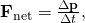where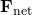is the net external force,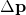is the change in momentum, andis the change in time.

Newton’s Second Law of Motion in Terms of Momentum

The net external force equals the change in momentum of a system divided by the time over which it changes.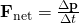Making Connections: Force and Momentum

Force and momentum are intimately related. Force acting over time can change momentum, and Newton’s second law of motion, can be stated in its most broadly applicable form in terms of momentum. Momentum continues to be a key concept in the study of atomic and subatomic particles in quantum mechanics.

This statement of Newton’s second law of motion includes the more familiar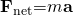as a special case. We can derive this form as follows. First, note that the change in momentumis given by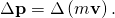If the mass of the system is constant, then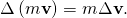So that for constant mass, Newton’s second law of motion becomes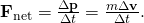Because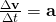, we get the familiar equationwhen the mass of the system is constant.

Newton’s second law of motion stated in terms of momentum is more generally applicable because it can be applied to systems where the mass is changing, such as rockets, as well as to systems of constant mass. We will consider systems with varying mass in some detail; however, the relationship between momentum and force remains useful when mass is constant, such as in the following example.

Calculating Force: Venus Williams’ Racquet

During the 2007 French Open, Venus Williams hit the fastest recorded serve in a premier women’s match, reaching a speed of 58 m/s (209 km/h). What is the average force exerted on the 0.057-kg tennis ball by Venus Williams’ racquet, assuming that the ball’s speed just after impact is 58 m/s, that the initial horizontal component of the velocity before impact is negligible, and that the ball remained in contact with the racquet for 5.0 ms (milliseconds)?

Strategy

This problem involves only one dimension because the ball starts from having no horizontal velocity component before impact. Newton’s second law stated in terms of momentum is then written as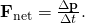As noted above, when mass is constant, the change in momentum is given by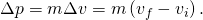In this example, the velocity just after impact and the change in time are given; thus, once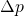is calculated,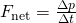can be used to find the force.

Solution

To determine the change in momentum, substitute the values for the initial and final velocities into the equation above.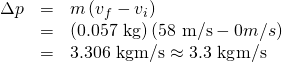Now the magnitude of the net external force can determined by using: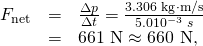where we have retained only two significant figures in the final step.

Discussion

This quantity was the average force exerted by Venus Williams’ racquet on the tennis ball during its brief impact (note that the ball also experienced the 0.56-N force of gravity, but that force was not due to the racquet). This problem could also be solved by first finding the acceleration and then using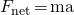, but one additional step would be required compared with the strategy used in this example.

# Section Summary

• Linear momentum (momentum for brevity) is defined as the product of a system’s mass multiplied by its velocity.
• In symbols, linear momentumis defined to be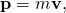where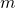is the mass of the system and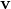is its velocity.

• The SI unit for momentum is.
• Newton’s second law of motion in terms of momentum states that the net external force equals the change in momentum of a system divided by the time over which it changes.
• In symbols, Newton’s second law of motion is defined to be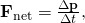is the net external force,is the change in momentum, andis the change time.

# Conceptual Questions

An object that has a small mass and an object that has a large mass have the same momentum. Which object has the largest kinetic energy?

An object that has a small mass and an object that has a large mass have the same kinetic energy. Which mass has the largest momentum?

Professional Application

Football coaches advise players to block, hit, and tackle with their feet on the ground rather than by leaping through the air. Using the concepts of momentum, work, and energy, explain how a football player can be more effective with his feet on the ground.

How can a small force impart the same momentum to an object as a large force?

# Problems & Exercises

(a) Calculate the momentum of a 2000-kg elephant charging a hunter at a speed of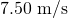. (b) Compare the elephant’s momentum with the momentum of a 0.0400-kg tranquilizer dart fired at a speed of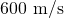. (c) What is the momentum of the 90.0-kg hunter running at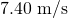after missing the elephant?

(a)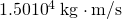(b) 625 to 1

(c)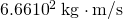(a) What is the mass of a large ship that has a momentum of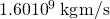, when the ship is moving at a speed of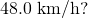(b) Compare the ship’s momentum to the momentum of a 1100-kg artillery shell fired at a speed of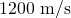.

(a) At what speed would a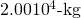airplane have to fly to have a momentum of(the same as the ship’s momentum in the problem above)? (b) What is the plane’s momentum when it is taking off at a speed of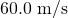? (c) If the ship is an aircraft carrier that launches these airplanes with a catapult, discuss the implications of your answer to (b) as it relates to recoil effects of the catapult on the ship.

(a)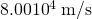(b)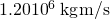(c) Because the momentum of the airplane is 3 orders of magnitude smaller than of the ship, the ship will not recoil very much. The recoil would be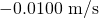, which is probably not noticeable.

(a) What is the momentum of a garbage truck that is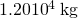and is moving at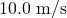? (b) At what speed would an 8.00-kg trash can have the same momentum as the truck?

A runaway train car that has a mass of 15,000 kg travels at a speed ofdown a track. Compute the time required for a force of 1500 N to bring the car to rest.

54 s

The mass of Earth is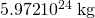and its orbital radius is an average of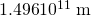. Calculate its linear momentum.

## Glossary

linear momentum
the product of mass and velocity
second law of motion
physical law that states that the net external force equals the change in momentum of a system divided by the time over which it changes